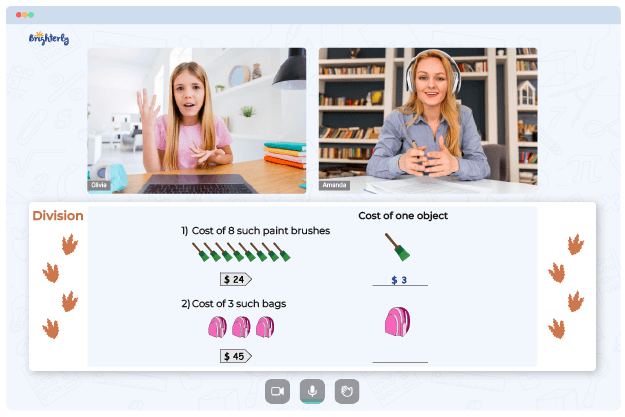# Factors And Multiples Worksheets

Factors and multiples worksheets are designed to help students learn factors and multiples.

A factor is a number that can divide another number completely without a remainder. Hence, if you’re asked to write down the factors of a given number, you’ll need to come up with a list of numbers that can divide the given number completely.

On the other hand, a multiple is the product when you multiply a number by another number. Therefore, if you’re asked to give the multiples of a given number, you need to list the numbers you get when you multiply the given number with other numbers.

Before you download our multiples and factors worksheets, below are the differences between factors and multiples and their benefits.

Math for Kids

Is Your Child Struggling With Math?
1:1 Online Math Tutoring## Difference Between Factors and Multiples

The following are the differences between factors and multiples:

 Factors Multiples They are numbers that can divide a given number completely They are the numbers you get when you multiply a given number by other numbers The factors of a number can be found through division The multiples of a given number are found through multiplication The factors of a given number are finite, which means they are limited and countable. The multiples of a given number are infinite, which means they are endless. The factors of a number are less than or equal to the number. They cannot be higher than the number The multiples of a number should be greater than or equal to the number. The multiples of a number cannot be lower than the number.

## Benefits of Multiple and Factor Worksheets### Factors And Multiples Worksheets PDF

Factors And Multiples Worksheet### Factors And Multiples Worksheets PDF

Least Common Multiple And Greatest Common Factor Worksheets### Factors And Multiples Worksheets PDF

Multiple And Factor Worksheets### Factors And Multiples Worksheets PDF

Multiple And Factors Worksheets

Multiple and factors worksheets will help elementary school kids improve their Math skills. The worksheet will help improve their speed and accuracy when calculating and doing number crunching.

After studying these multiple and factor worksheets, students should understand the concept of factors and multiples. They will learn how to identify and list the factors of a given number. Students will also learn how to find the multiples of a number.

You can also download the least common multiple and greatest common factor worksheets below.

### More Worksheets

Troubles with Division?• Does your child need extra help with mastering of division?
• Start studying with an online tutor.

Is your child finding it hard to master division lessons? An online tutor could be of assistance.